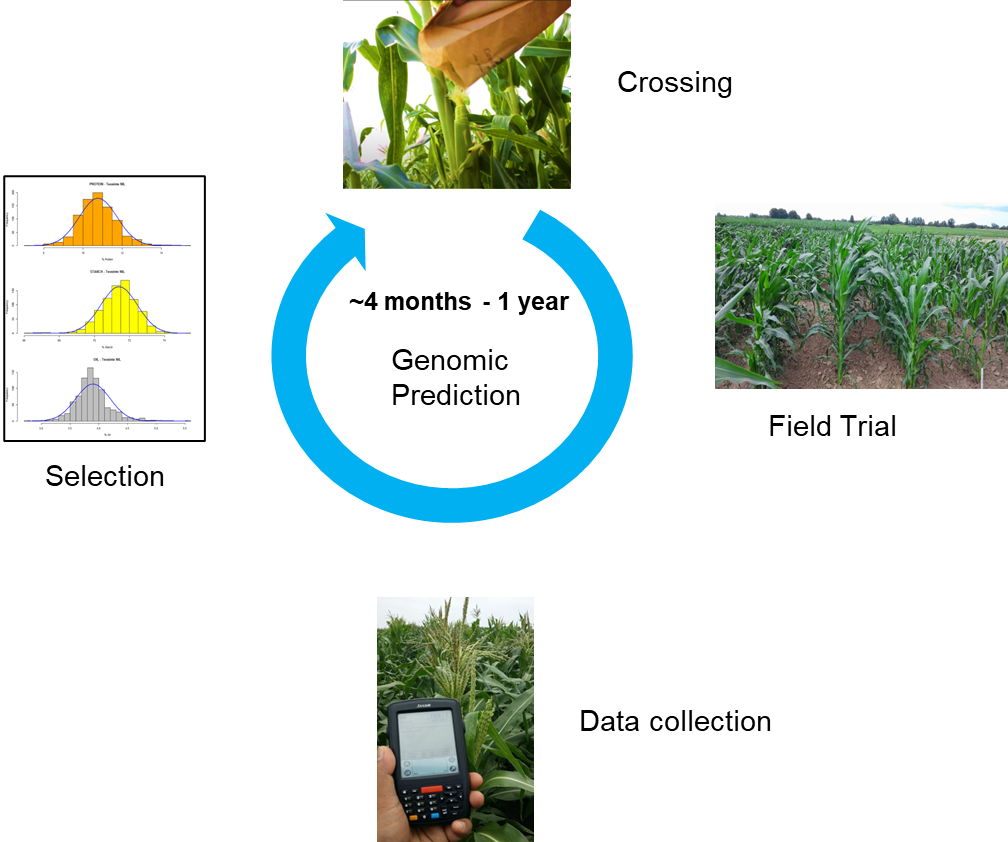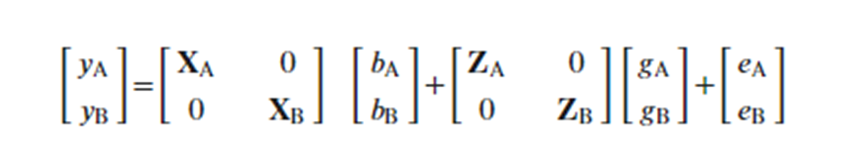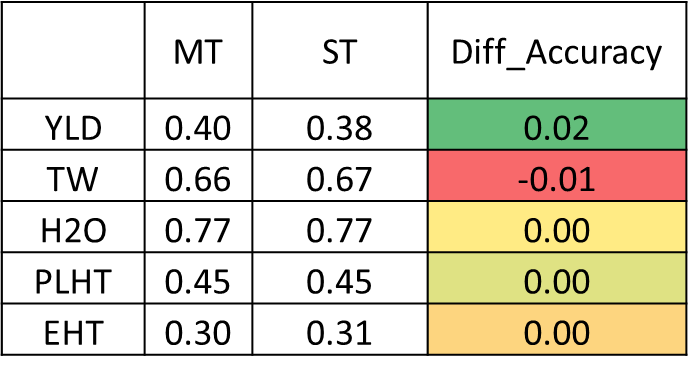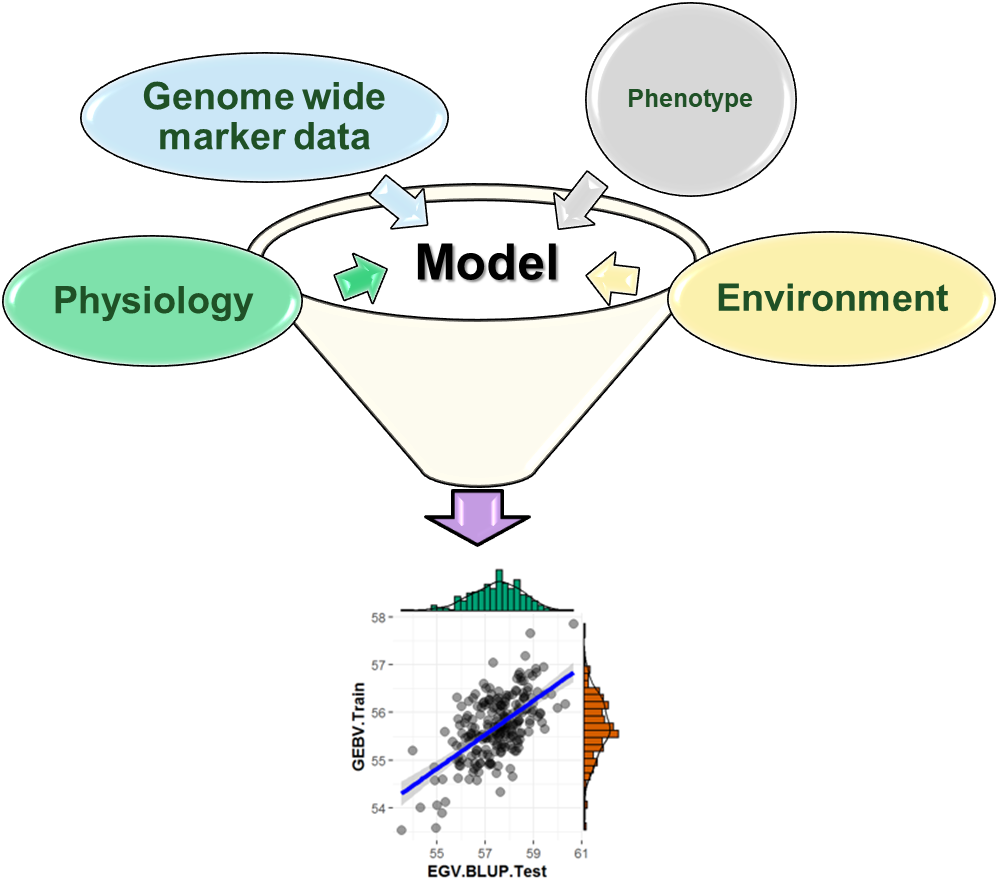Meuwissen et al 2000 first proposed genomic selection to predict breeding values in animals and plants – the idea was to use DNA markers as predictors in model development to estimate marker effects to predict genomic estimated breeding values. Genomic selection (GS) is becoming a popular technique enabling breeders to select lines using genome-wide marker data before estimating their actual performance in the fields.Genomic prediction models focus on accurately predicting the breeding value by simultaneously modeling all the markers contributing to prediction accuracy.

Breeding value = ∑wi * βi

The goal is to accurately predict the breeding values (BV). Individuals with genotypic and phenotypic information are used to model relationships between phenotype and genotype of observed lines, and then the model enables the predictions of phenotypes for unobserved lines using their marker profile. That is, in association mapping, population structure can confound marker effect estimates, and rapid LD decay allows for more precise mapping, however, in genomic prediction population structure can be utilized to increase prediction accuracy, therefore, more closely related prediction and training sets tend to yield better prediction results.

There are many factors that affect the prediction accuracy of the breeding values:

• Genetic architecture of the trait
• LD between markers
• Related among individual in training and test set
• Heritability
• Marker Density
• Training set size and composition
• GS models
• Genomic best linear unbiased predictor (GBLUP)
• Bayesian models
• Random Forest
• Support vector machine

## Uni-Trait (UT GS) or Single Trait GS Model

Most of the GS models evaluated in plant breeding have been unitrait (UT), where single traits are predicted using the below equation:

𝑦 = μ + 𝑍𝑔 + 𝑒

Where:

• y: Phenotype of interest (e.g., Grain Yield)
• μ: Overall mean
• 𝑍: Design matrix linking genotypes to breeding values
• g: vector of genomic breeding values
• e: random residuals

## Multi-Trait GS model

Recently, breeders have moved to adopt Multi-Yrait (MT) GS models because predictions are required for multiple traits (e.g yield, test weight, moisture, plant and ear height) simultaneously, and their combination of may improve prediction accuracies.

• Jia and Jannink (2012) show that prediction accuracy improved for primary traits with low heritability in barley (Hordeum vulgare L.) when a secondary correlated trait is used in MT-GS models.
• Sun et al. (2019) demonstrated the increase in GS prediction accuracy for wheat (Triticum aestivum L.) grain yield when secondary traits such as normalize difference vegetation index (NDVI) and canopy temperature were included in MT-GS models.
• Bhatta et al. (2020) compared UT- and MT-GS models for predicting quality traits in barley and concluded that MT-GS models have better performance under within- and across-environment predictions.

MT-GS model leverage on the genetic information between correlated traits to predict various traits simultaneously using same set of predictors.Where:

• yA and yB: BLUPs of primary and secondary traits
• X and Z : matrix for fixed and random effects
• b: Vector of means for primary and secondary traits
• g: Random genetic effects
• e: residuals for each trait

## MT-GS in R using BGLR package

In this tutorial blog post, I compared single-trait and multi-trait GS models utilizing multi-year (6 years) and location phenotype data and genotype data consisting ~10K markers. In the analysis, Yield, Test weight, Moisture, and Plant- & Ear height were the evaluated traits. And unfortunately due to data protection issue - i am uable to share the raw data used in the analysis.

All the analyses were done in R with below paramteres:

• BGLR-R package (Perez & de los Campos, 2014)
• Model evaluation: Training (n=3654) and Test sets (n=300)
• Multi-trait prediction using Single Vector Decomposition (SVD)
• 12,000 Iterations
• Single trait prediction using GBLUP

## R Code for MT & ST GS using BGLR package

Note: The below R codes were modified from the original code obtained from the authors of BGLR package, which is available at this link https://github.com/gdlc/BGLR-R/blob/master/inst/md/MULTITRAIT.md


setwd("~/My_Projects/year_2022/seminar_QuantGen/R2g2_MT_GS/molecularData_r1g1_male_csv")

## Deriving Eigenvectors of the phenotype matrix

rm(list = ls())

library(BGLR)

# A phenotype matrix with 4 traits

Y2 <- as.matrix(Y2)

Y2 <- scale(Y2)

# Singular value decompositions Y=UDV' Singular Value Decomposition of a Matrix

SVD = svd(Y2)
U=SVD$u D=diag(SVD$d)
V=SVD$v # Molecular markers X <- read.csv("numeric2_geno_r1g1_male.csv", check.names = F, header = T) X <- scale(X) ## regression coefficients B=matrix(nrow=ncol(X),ncol=ncol(Y2)) ETA=list(list(X=X,model='BayesB')) for(i in 1:ncol(Y2)){ fm=BGLR(y=U[,i],ETA=ETA,verbose=F, nIter = 12000, burnIn = 2000) #use more iterations! B[,i]=fm$ETA[]$b } # Rotating coefficients to put them in marker space BETA=B%*%D%*%t(SVD$v)

# Prediction
YHat=X%*%BETA

# correlation in training
for(i in 1:ncol(Y2)){ print(cor(Y2[,i],YHat[,i])) }

#Training-testing comparing single and multi-trait

## Training/Testing partition

set.seed(195021)
N=nrow(X)
tst=sample(1:N,size=300)
Y.TRN=Y2[-tst,]
Y.TST=Y2[tst,]

X.TST=X[tst,]

ETA=list(list(X=X[-tst,],model='BayesB'))

## Prediction using single-trait models

YHAT.ST=matrix(nrow=nrow(Y.TST),ncol=5)

for(i in 1:5){
fm=BGLR(y=Y.TRN[,i],ETA=ETA,verbose=F, nIter = 12000, burnIn = 2000) #use more iterations!
YHAT.ST[,i]=X.TST%*%fm$ETA[]$b
}

## Prediction using eigenvectors aka MT models ??

SVD=svd(Y.TRN)
U=SVD$u D=diag(SVD$d)
V=SVD$v B=matrix(nrow=ncol(X), ncol=ncol(U)) for(i in 1:5){ fm=BGLR(y=U[,i],ETA=ETA,verbose=F , nIter = 12000, burnIn = 2000) #use more iterations! B[,i]=fm$ETA[]\$b
}

BETA=B%*%D%*%t(V)

YHAT.EV=X.TST%*%BETA

## Comparison

for(i in 1:5){
message( round(cor(Y.TST[,i],YHAT.ST[,i]),3),"   ",round(cor(Y.TST[,i],YHAT.EV[,i]),3))
}



## TABLE: Independent predictions with uni- and multitrait genomic selection modelsBased on my preliminary analysis, MT-GS models had similar prediction accuracies as UT-GS models for most of the evaluated triats, however, prediction accuracy of the grain yield was about 2% higher with MT-GS models, which was interesting, since it is well known that yield is a complex trait with low heritability.

## Opportunities to improve predictive accuracy of complex traits using multi-trait modelsRogers et al 2022 demonstrated that addition of environmental data as a measure of similarity between environments aids in environment-specific genomic prediction. Also, G × E modeling will likely be most useful for programs that have a set of target environments for future lines that are looking to direct early stage material toward a specific target population of environments.

Therefore, physiological and envrionmental data such as Canopy temperature, Normalized difference vegetation index (NDVI), Soil profiles, Rainfall, Temperaturecan be used to determine water status and biomass of plants, and or possiblly improve the prediction accuracy of quantitative traits as well as help explain the unknown variance component that is ignored in single trait GS models..

Thank you for reading this tutorial. I really hope these steps will assist in your analysis. If you have any questions or comments, please comment below or send an email.

Pérez P, de los Campos G. Genome-wide regression and prediction with the BGLR statistical package. Genetics. 2014 Oct;198(2):483-95. https://doi.org/10.1534/genetics.114.164442 Epub 2014 Jul 9. PMID: 25009151; PMCID: PMC4196607.

Jia, Y., & Jannink, J. L. (2012). Multiple-trait genomic selection methods increase genetic value prediction accuracy. Genetics, 192, 1513–1522. https://doi.org/10.1534/genetics.112.144246

Lozada, D. N., & Carter, A. H. (2019). Accuracy of single and multi-trait genomic prediction models for grain yield in US Pacific Northwest winter wheat. Crop Breeding, Genetics and Genomics, 1, e190012. https://doi.org/10.20900/cbgg20190012

Bhatta, M., Gutierrez, L., Cammarota, L., Cardozo, F., Germán, S., Gómez-Guerrero, B., Pardo, M. F., Lanaro, V., Sayas, M., & Castro, A. J. (2020). Multi-trait genomic prediction model increased the predictive ability for agronomic and malting quality traits in barley (Hordeum vulgare L.). G3: Genes, Genomes, Genetics, 10, 1113–1124. https://doi.org/10.1534/g3.119.400968

Sun, J., Poland, J. A., Mondal, S., Crossa, J., Juliana, P., Singh, R. P., Rutkoski, J. E., Jannink, J. L., Crespo-Herrera, L., Velu, G., Huerta-Espino, J., & Sorrells, M. E. (2019). High-throughput phenotyping platforms enhance genomic selection for wheat grain yield across populations and cycles in early stage. Theoretical and Applied Genetics, 132, 1705–1720. https://doi.org/10.1007/s00122-019-03309-0

Rogers, A. R., & Holland, J. B. (2022). Environment-specific genomic prediction ability in maize using environmental covariates depends on environmental similarity to training data. G3, 12(2), jkab440. https://doi.org/10.1093/g3journal/jkab440